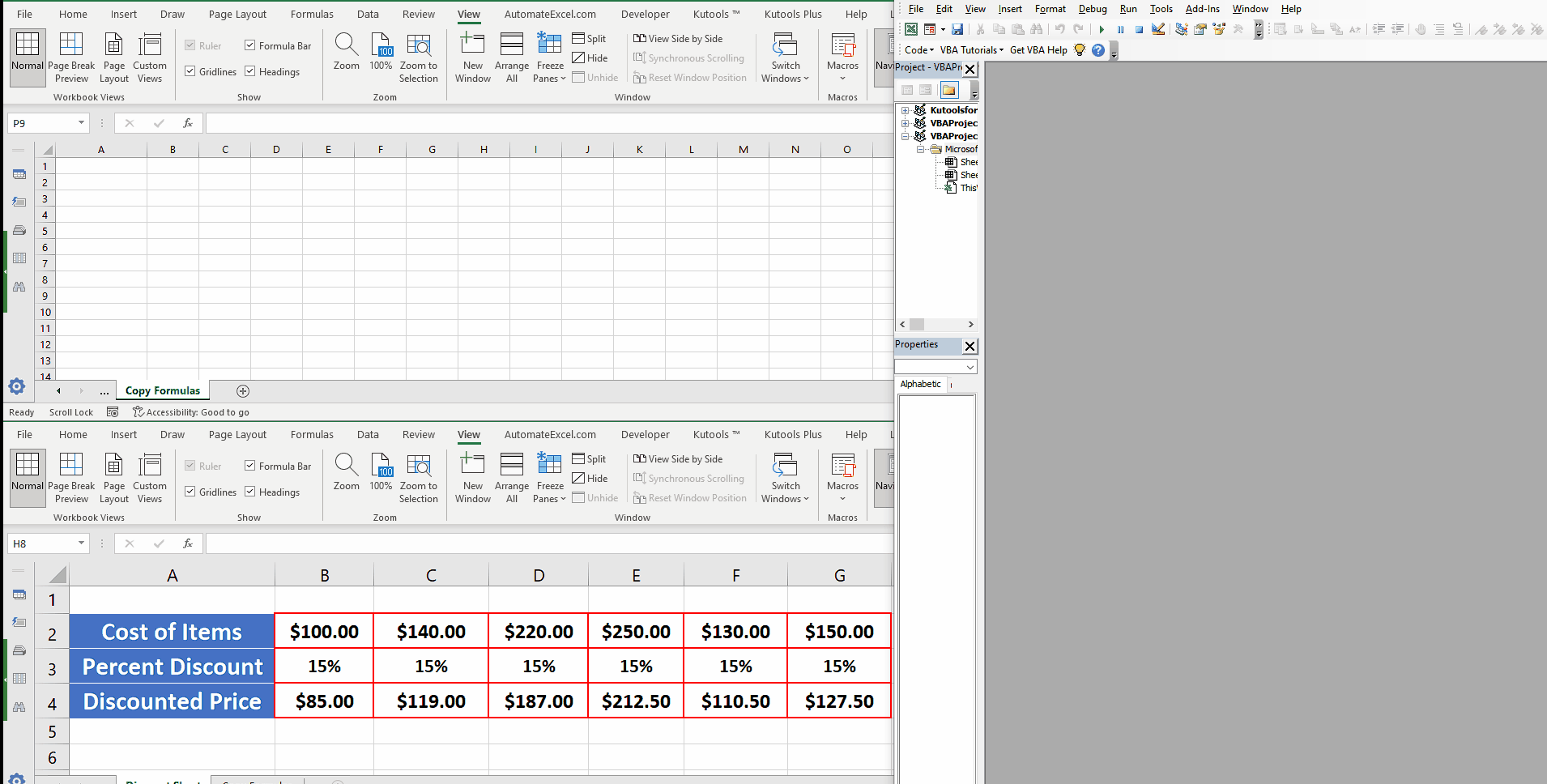# How to copy formulas to another sheet using VBA in Excel

In this tutorial we’ll learn how to copy formulas from one sheet to another sheet using the power of VBA. Let’s look at our workbook first. This will help us to understand the code better. Our workbook contains two sheets. The first sheet named “Discount Sheet” contains the data and formulas that we wish to copy to the “Copy Formulas” sheet.Excel is a very powerful tool to perform mathematical and statistical calculations on numeric data. Excel supports Visual Basic for Applications also known as VBA. We can write from simple to complex pieces of code to automate various functions in Excel through VBA.

### Step 1 – Open the VBA Editor by pressing ALT+F11 or through Developer Tab

– In Excel’s main menu, click on the Developer tab and then click on Visual Basic.

### Step 2 – Insert the Module, write code to copy formulas and run the code

– The previous step will open the VBA editor. In this editor’s main menu tabs, click on Insert —-> Module.
– This will insert a blank module in the editor where we can write the code to copy formulas from the “Discount Sheet” which contains the data and formulas to the “Copy Formulas” sheet. We’ll use Range.Copy & Range.PasteSpecial methods to achieve our goal. The code snippet is available at the end of the document.

## The Code Snippet

This is the code used in this tutorial.

Here the sub procedure Copy_Range_withFormula_ToAnother_Sheet() is the main procedure which is doing the work.

The first highlighted line Sheets(“Discount Sheet”).Range(“A2:G4”).Copy copies the data from the range “A2:G4” of “Discounted Sheet”.

Then the second highlighted line

Sheets(“Copy Formulas”).Range(“A2”).PasteSpecial Paste:=xlPasteFormulasAndNumberFormats, _ Operation:=xlNone, SkipBlanks:=False, Transpose:=False

pastes the copied data to the other sheet named “Copy Formulas”. We used Range.PasteSpecial method along with Paste Type xlPasteFormulasAndNumberFormats to tell Excel to paste the copied data along with all formulas and number formats as well.